Using Computing in Mathematics Courses

Author: Jim Carlson
Date: March 1, 1995

Below we give two examples of how computing can be used in mathematics courses. The first is to find the equilibrium temperature distribution of a thin metal plate. The physical principles are easily explainable, and from these one can set up a mathematical model of the plate and solve it by solving a (large) system of linear equations. The second example is a model of population dynamics.

The particular computing tool we use is Maple. However, any good general-purpose tool could be used.

Courses

Below is a partial list of elmentary courses which make regular use of computing:

Temperature at Equilibrium

The Physics

The temperature distribution of a thin conducting plate insulated on both sides is determined by the temperature along the edges. The physics of the plate is governed by the mean value principle:

The temperature at a given point is the same as the average temperature of nearby points.
This expresses a kind of balance between inflows and outflows of heat: a point at a temperature lower than the local average temperature will warm up; a point at a temperature higher than the local average will cool down.

The Mathematics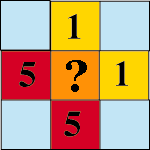One way to express the mean value principle in mathematical form is to divide the plate into a grid of small square cells, as in the figure on the right. Then

The temperature of a cell is one-quarter the sum of the temperatures of the four nearest neighbors.
For our example this gives the temperature of the middle cell as
T = (1 + 1 + 5 + 5)/4 = 3

When there is just one interior cell, as in the three-by-three grid above, simple arithmetic is enough to solve the problem using the mean value principle. When the grid is larger, say, four-by-four, as in the figure below, the mean value principle yields a system of four equations in four unknowns.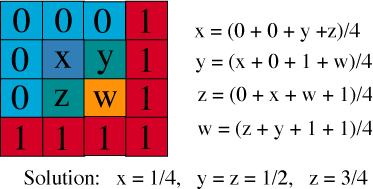In general there is one equation for each interior cell. For example, in the figure below we have an eight-by-eight grid, hence a six-by-six grid of interior cells. Consequently the mean-value principle yields a system of thirty-six equations in thirty-six unknowns.The Computation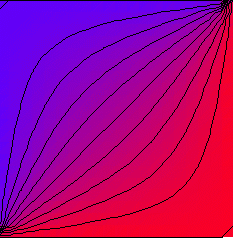On the one hand an eight-by-eight grid is too small in the sense that it does not give a grid fine enough to determine the interior temperatures with accuracy. On the other hand it is too large in the sense that thirty-six equations is already too large to be solved by hand. However, with a computer we can solve large systems and so resolve the dilemma. The first task is to find an efficient way of writing the equations. If T[i,j] denotes the temperature of the cell in the i-th row and the j-th column, then we have

T[i,j] = (1/4)( T[i-1,j] + T[i+1,j] + T[i,j-1] + T[i,j+1] )
Because the notation is so systematic, we can use a computer program both to write the equations and to solve them using, for example, the method of elimination discovered by Gauss (1777-1855).

The figure below illustrates what happens when the plate is divided into a 22-by-22 grid with temperature T = 0 along the top and left and temperature T = 1 along the bottom and right. To determing the interior temperatures we solve a system of 400 equations in 400 unknowns. Since it is difficult to interpret a table of 400 numbers we represent the result graphically, coding the numerical temperature by a color between red and blue, where red is the hottest temperature and blue is the coldest. We can also use the computer to trace the isotherms --- the lines along which temperature is constant.

Population Dynamics

Consider a community of insects whose population at time t = n is x_n. A simple model for the dynamics of this population assumes that the population at time t = n+1 is completely determined by the population at time t = n. In other words, x_{n+1} is a function of x_n, a fact we can write as
x_{n+1} = f(x_n)
This choice for the next-state function f is
f(x) = kx
For example, if k = 2, then
x_n = 2^nx_0
This kind of function models bacterial populations as long as the bacteria are not too crowded. Growth is exponential, and quite rapid. For example, if the unit of time is four hours, then the sequence of populations for the first day is
1, 2, 4, 8, 16, 32
For the first week it is (in round numbers)
32; 1,000;  30,000;  1,000,000;  30,000,000; 1,000,000,000; 30,000,000,000
Such growth, illustrated in the figure below, cannot continue for long, so a more realistic model is needed.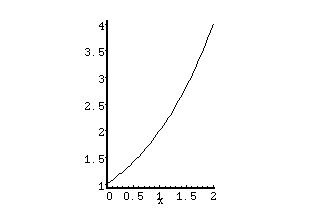We will examine the model introduced by the Princeton biologist Robert May in his study of insect populations. The idea is to use quadratic functions

f(x) = kx(1-x)
Unfortunately, there is no simple formula for the population sequence { x_n }, so we must use other tools to analyze its behavior. One approach is to generate the sequence using a simple computer program, then graph the results. When the initial population is 0.1 units and k = 2, we get this:
.1, .18, .2952,  .4161, .4859, .4996, .4999, .5000, .5000, ...
It is clear what happens: the population quickly approaches an "equilibrium population" of 0.5 units. The equilibrium value is in fact a solution of the quadratic equation
x = f(x)
It is a fixed point of the function. A graph of population versus time looks like this: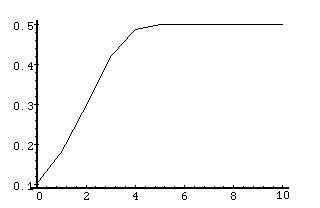This is how many populations behave: there is rapid approach to equilibrium. For example, if we start above equilibrium, we have something like the graph below.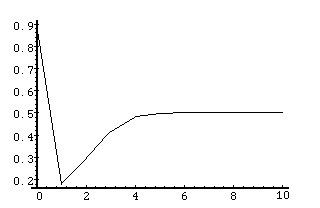This is pretty tame. However, as k increases, i.e., as the fecundity of the population increases, thing become more interesting. Here is what we find when k = 3.2: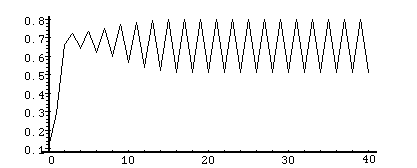The population rapidly settles into a cycle of period 2. Let's see what happens when the k = 3.5: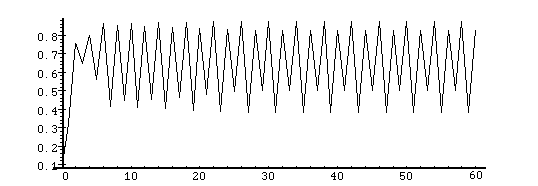The eventual dynamic is cyclic, but with double the previous one: each four years the pattern repeats itself. It is worth exploring the behavior for other values of k. Here is what we find for k = 4: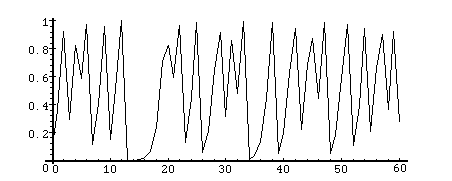The behavior is chaotic. This means several things. First, small changes in initial conditions lead to large changes in the population level for a fixed time in the "distant" future. These changes do not follow a simple pattern and so are "unpredictable". Second, there is no discernible periodicity. This is rather natural, since as k gets closer to 4, the period gets larger and larger, i.e., tends to infinity.

May's model shows that very simple, deterministic systems can generate extremely complicated behavior, behavior which is sometimes so sensitive to initial conditions that it cannot be predicted from measured data in any realistic way.